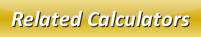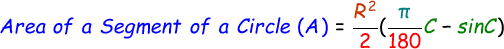# Area of a Segment of a Circle Calculator

Segment of a circle is the region of a circle which is bounded by arc and chord of a circle.

We can find the area of a sector of a circle with the help of this below formula:where,
R = Radius of the circle
C = Central Angle [degrees]

Use our below online area of a sector of a circle calculator by entering the the radius and the central angle of the circle in the respective input boxes and click calculate to get the output.

 Radius of the Circle (R): Central Angle (C): [degrees] Area of a Segment of a Circle (A):

Latest Calculator Release

Average Acceleration Calculator

Average acceleration is the object's change in speed for a specific given time period. ...

Free Fall Calculator

When an object falls into the ground due to planet's own gravitational force is known a...

Torque Calculator

Torque is nothing but a rotational force. In other words, the amount of force applied t...

Average Force Calculator

Average force can be explained as the amount of force exerted by the body moving at giv...

Angular Displacement Calculator

Angular displacement is the angle at which an object moves on a circular path. It is de...